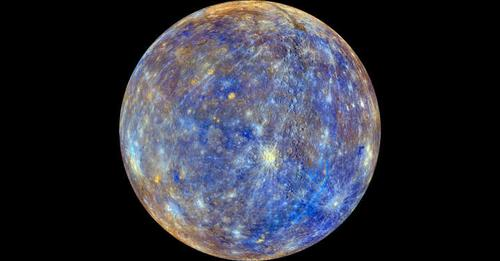## Post per PageThe order of the planets is something most of us learn in school: Mercury, Venus, Earth, Mars, Jupiter, Saturn, Uranus, Neptune, and (until 2006) Pluto.

So, you would be forgiven for thinking that as Earthlings, our closest planetary neighbor is Venus. And in a way, you would be right – at its nearest, Venus approaches Earth closer than any other planet in the Solar System. Likewise, its orbit is closer to our orbit than any other. However, in another sense, you would be wrong. At least, that is the argument put forward in an article published in Physics Today.

To identify our closest neighbor, engineers affiliated with NASA, Los Alamos National Observatory, and the US Army's Engineer Research Development Center built a computer simulation to calculate the average proximity of Earth to its three nearest planets (Mars, Venus, and Mercury) over a 10,000-year-period. Because of the way the planets align during their respective orbits, the model shows that Earth spends more time nearer to Mercury than either Venus or Mars.

“In other words, Mercury is closer to Earth, on average, than Venus is because it orbits the Sun more closely,” the authors explain.

Indeed, it’s not just Earth. Further calculations suggest that all seven planets in the Solar System (minus Mercury) spend most of their orbit closer to “the Winged Messenger” than any other planet. Sounds impossible? This is how they worked it out.

The results are based on a technique called the point-circle method (PCM) – in essence, a mathematical equation that takes the orbits of two planets as circular, concentric, and coplanar, and calculates the average distance between two planets as they orbit the sun.

"From the PCM, we noticed that the distance between two orbiting bodies is at a minimum when the inner orbit is at a minimum," the authors explain.

"That observation results in what we call the whirly-dirly corollary (named after an episode of the cartoon Rick and Morty): For two bodies with roughly coplanar, concentric, circular orbits, the average distance between the two bodies decreases as the radius of the inner orbit decreases."

"It’s clear from this corollary, and from the table, that Mercury (average orbital radius of 0.39 AU), not Venus (average radius of 0.72 AU), is the closest planet to Earth on average." (AU is astronomical units, the distance between Earth and the Sun.)

To test their hypothesis, they built a computer simulation that tracked the positions of all four planets over a 10,000-year-period and calculated the average distance between them. The results of this simulation differed from traditional calculations (determined by subtracting the average radius of the inner orbit from the average radius of the outer orbit) by a staggering 300 percent. Yet differed from the PCM calculations by a relatively insignificant 1 percent.

It found that the average distance between Earth and Venus was 1.136 astronomical units (0.28 on the “old method”). In comparison, the average distance between Earth and Mercury was 1.039 astronomical units (0.61 on the “old method”).

The hypothesis has yet to be submitted to a peer-reviewed paper and will no doubt be put through a thorough cross-examination by experts in the field, but the authors have already noted some possible uses for their newly-devised PCM equation.

"With the right assumptions, PCM could possibly be used to get a quick estimate of the average distance between any set of orbiting bodies," the authors write.

"Perhaps it can be useful for quickly estimating satellite communication relays, for which signal strength falls off with the square of distance. In any case, at least we know now that Venus is not our closest neighbor – and that Mercury is everybody’s."

[H/T: Physics Today]